# SCERT Maharashtra Question Bank solutions for 10th Standard SSC Science and Technology 1 Maharashtra State Board 2021 chapter 9 - Carbon compounds [Latest edition]

#### Chapters## Chapter 9: Carbon compounds

Choose the correct option.Find the correlation.Find odd one out.Answer in one sentence.Match the columnsRight or Wrong sentenceWrite an ExplanationWrite scientific reasonsSolve the following QuestionsDistinguish betweenWrite short notesAnswer the FollowingAnswer the following questions
Choose the correct option.

### SCERT Maharashtra Question Bank solutions for 10th Standard SSC Science and Technology 1 Maharashtra State Board 2021 Chapter 9 Carbon compounds Choose the correct option.

#### Choose the correct option.

Choose the correct option. | Q 1

Generally, the melting and boiling point of carbon compounds are found to be less than _______ °C.

• 300

• 100

• 200

• 150

Choose the correct option. | Q 2

Number of valence electrons in a carbon atom is _______.

• 4

• 5

• 1

• 3

Choose the correct option. | Q 3

The bond between two oxygen atoms is _______ bond.

• double

• triple

• single

• none of these

Choose the correct option. | Q 4

The molecular masses of a carbon compound spread over a range of _______.

• 1012

• 1014

• 1010

• 1013

Choose the correct option. | Q 5

The unsaturated hydrocarbons containing a carbon-carbon double bond are called _______.

• Alkenes

• Alkanes

• Alkynes

• Alcohol

Choose the correct option. | Q 6

The phenomenon in which compounds having different structural formulae have the same molecular formula is called _______.

• structural isomerism

• catenation

• homologous

• functional group

Choose the correct option. | Q 7

From the following hydrocarbon _______ is the cyclic hydrocarbon.

• isobutane

• propyne

• benzene

• isobutylene

Choose the correct option. | Q 8

While going in an increasing order there is a rise in the molecular mass of the consecutive members of the homologous series by _______.

• 14 u

• 15 u

• 16 u

• 17 u

Choose the correct option. | Q 9

The general molecular formula for the homologous series of alkynes is _______.

• CnH2n

• "C"_"n""H"_("2n" + 2)

• "C"_"n""H"_("2n" - 2)

• "C"_"n""H"_("2n" - 1)

Choose the correct option. | Q 10

_______ is one of the combustible components of L.P.G.

• Methane

• Ethane

• Propane

• Butanol

Choose the correct option. | Q 11

At room temperature ethanol is _______.

• solid

• gas

• plasma

• liquid

Choose the correct option. | Q 12

Generally _______ is called spirit.

• methanol

• ethanol

• propanol

• butanol

Find the correlation.

### SCERT Maharashtra Question Bank solutions for 10th Standard SSC Science and Technology 1 Maharashtra State Board 2021 Chapter 9 Carbon compounds Find the correlation.

#### Find the correlation.

Find the correlation. | Q 1

Solid : iodine : : _______ : bromine

Find the correlation. | Q 2

CH3–CH2–CHO : propanal : : CH3–COOH : _______

Find the correlation. | Q 3

Ketone : –CO– : : Ester : _______

Find the correlation. | Q 4

Cyclohexane : Cyclic hydrocarbon : : Isobutylene : _______

Find the correlation. | Q 5

Saturated hydrocarbon : Single bond : : Unsaturated hydrocarbon : _______

Find the correlation. | Q 6

Saturated carbon compounds : blue flame : : Unsaturated carbon compounds : _______

Find odd one out.

### SCERT Maharashtra Question Bank solutions for 10th Standard SSC Science and Technology 1 Maharashtra State Board 2021 Chapter 9 Carbon compounds Find odd one out.

Find odd one out. | Q 1

Find the odd one out and give its explanation.

• Ethylene

• Styrene

• Propylene

• Teflon

Find odd one out. | Q 2

Find the odd one out and give its explanation.

• Butane

• Methane

• Benzene

• Ozone

Find odd one out. | Q 3

Find the odd one out and give its explanation.

• CH4

• C2H6

• C5H12

• CaCO3

Find odd one out. | Q 4

Find the odd one out and give its explanation.

• C2H2

• C3H8

• C2H6

• CH4

Find odd one out. | Q 5

Find the odd one out and give its explanation.

• C2H4

• C4H10

• C3H8

• CH4

### SCERT Maharashtra Question Bank solutions for 10th Standard SSC Science and Technology 1 Maharashtra State Board 2021 Chapter 9 Carbon compounds Answer in one sentence.

#### Answer the following question in one sentence.

Answer in one sentence. | Q 1.1

Write the molecular formula of the given compound.

Ethyl ethanoate

Answer in one sentence. | Q 1.2

Write the molecular formula of the given compound.

Sodium ethanoate

Answer in one sentence. | Q 1.3

Write the molecular formula of the given compound.

Sodium ethoxide

Answer in one sentence. | Q 1.4

Write the molecular formula of the given compound.

Stearic acid

Answer in one sentence. | Q 1.5

Write the molecular formula of the given compound.

Oleic acid

Answer in one sentence. | Q 1.6

Write the molecular formula of the given compound.

Palmitic acid

Answer in one sentence. | Q 2.01

Write the molecular formula of the given compound.

Ethylene

Answer in one sentence. | Q 2.02

Write the molecular formula of the given compound.

Benzene

Answer in one sentence. | Q 2.03

Write the molecular formula of the given compound.

Acetic acid

Answer in one sentence. | Q 2.04

Write the molecular formula of the given compound.

Propylene

Answer in one sentence. | Q 2.05

Write the molecular formula of the given compound.

Acetylene

Answer in one sentence. | Q 2.06

Write the molecular formula of the given compound.

Ethyl alcohol

Answer in one sentence. | Q 2.07

Write the molecular formula of the given compound.

Acetone

Answer in one sentence. | Q 2.08

Write the molecular formula of the given compound.

Propene

Answer in one sentence. | Q 2.09

Write the molecular formula of the given compound.

Ethanol

Answer in one sentence. | Q 2.1

Write the molecular formula of the given compound.

Ethanoic acid

Answer in one sentence. | Q 2.11

Write the molecular formula of the given compound.

Isobutane

Answer in one sentence. | Q 3.1

Draw electron dot structure and line structure for a given molecule.

Hydrogen

Answer in one sentence. | Q 3.2

Draw electron dot structure and line structure for a given molecule.

Oxygen

Answer in one sentence. | Q 3.3

Draw electron dot structure and line structure for a given molecule.

Methane

Answer in one sentence. | Q 3.4

Draw electron dot structure and line structure for a given molecule.

Nitrogen

Answer in one sentence. | Q 3.5

Draw electron dot structure and line structure for a given molecule.

Ethene

Match the columns

### SCERT Maharashtra Question Bank solutions for 10th Standard SSC Science and Technology 1 Maharashtra State Board 2021 Chapter 9 Carbon compounds Match the columns

Match the columns | Q 1

Match the pairs.

 Group 'A' Group 'B' a. C2H6 1. Unsaturated hydrocarbon b. C2H2 2. Molecular formula of an alcohol c. CH4O 3. Saturated hydrocarbon d. C3H6 4. Triple bond
Match the columns | Q 2

Match the columns.

 Group A Group B 1. Straight chain hydrocarbon a) Benzene 2. Branched chain hydrocarbon b) Propyne 3. Cyclic hydrocarbon c) Isobutylene
Match the columns | Q 3

Match the columns.

 Group A Group B 1) Ether a) –OH 2) Ketone b) –O– 3) Ester c) –CO– 4) Alcohol d) –COO
Right or Wrong sentence

### SCERT Maharashtra Question Bank solutions for 10th Standard SSC Science and Technology 1 Maharashtra State Board 2021 Chapter 9 Carbon compounds Right or Wrong sentence

Right or Wrong sentence | Q 1

The number of electrons in the valence shell of a carbon atom is 4.

• Right

• Wrong

Right or Wrong sentence | Q 2

• Right

• Wrong

Right or Wrong sentence | Q 3

Carbon compounds contain only open chains of carbon atoms.

• Right

• Wrong

Right or Wrong sentence | Q 4

In general, saturated compounds are more reactive than unsaturated compounds.

• Right

• Wrong

Right or Wrong sentence | Q 5

Benzene is a cyclic unsaturated hydrocarbon.

• Right

• Wrong

Right or Wrong sentence | Q 6

Cyclohexane is a branched chain type of hydrocarbon.

• Right

• Wrong

Right or Wrong sentence | Q 7

As one ascends in any homologous series, physical properties change gradually.

• Right

• Wrong

Right or Wrong sentence | Q 8

There are different general molecular formula for all members of the homologous series.

• Right

• Wrong

Right or Wrong sentence | Q 9

In LPG, butane is a flammable component.

• Right

• Wrong

Right or Wrong sentence | Q 10

Substances that can give oxygen to other substances are called reductant.

• Right

• Wrong

Right or Wrong sentence | Q 11

Potassium permanganate is an oxidizing compound in regular use.

• Right

• Wrong

Right or Wrong sentence | Q 12

Colorless ethanol is in liquid state at room temperature.

• Right

• Wrong

Right or Wrong sentence | Q 13

Ethanol is soluble in water in all proportions.

• Right

• Wrong

Right or Wrong sentence | Q 14

Ester is a sweet-smelling compound.

• Right

• Wrong

Right or Wrong sentence | Q 15

Two carbon atoms can always form one or two covalent bonds.

• Right

• Wrong

Write an Explanation

### SCERT Maharashtra Question Bank solutions for 10th Standard SSC Science and Technology 1 Maharashtra State Board 2021 Chapter 9 Carbon compounds Write an Explanation

Write an Explanation | Q 1

Write an Explanation.

Alkane

Write an Explanation | Q 2

Write an Explanation.

Alkene

Write an Explanation | Q 3

Write an Explanation.

Alkyne

Write scientific reasons

### SCERT Maharashtra Question Bank solutions for 10th Standard SSC Science and Technology 1 Maharashtra State Board 2021 Chapter 9 Carbon compounds Write scientific reasons

Write scientific reasons | Q 1

Write scientific reason.

Ethylene is an unsaturated hydrocarbon.

Write scientific reasons | Q 2

Write scientific reason.

The flame appears yellow in the ignition of naphthalene.

Write scientific reasons | Q 3

Write scientific reason.

The color of iodine disappears in the reaction between vegetable oil and tincture iodine.

Write scientific reasons | Q 4

Write scientific reason.

Vegetable ghee is formed from the hydrogenation of vegetable oil in presence of nickel catalyst.

Write scientific reasons | Q 5

Write scientific reason.

Carbon has the property of forming many compounds.

Write scientific reasons | Q 6

Write scientific reason.

Benzene compounds are called aromatic compounds.

Solve the following Questions

### SCERT Maharashtra Question Bank solutions for 10th Standard SSC Science and Technology 1 Maharashtra State Board 2021 Chapter 9 Carbon compounds Solve the following Questions

Solve the following Questions | Q 1

Explain the Catenation power.

Solve the following Questions | Q 2

Explain covalent bond with example.

Solve the following Questions | Q 3

Explain the Structural isomerism term with example.

Solve the following Questions | Q 4.1

What is an oxidising agent?

Solve the following Questions | Q 4.2

Explain the following term with example.

Reduction

Solve the following Questions | Q 5

Explain the concept of heteroatoms with the help of examples.

Solve the following Questions | Q 6.1

Explain the following reaction with example.

Solve the following Questions | Q 6.2

Explain the following reaction with an example.

Substitution reaction

Solve the following Questions | Q 6.3

Explain the following reaction with an example.

Esterification

Solve the following Questions | Q 6.4

Explain the following reaction with an example.

Saponification

Solve the following Questions | Q 7.1

What are catalysts?

Solve the following Questions | Q 7.2

Give a chemical reaction in which a catalyst is used.

Solve the following Questions | Q 8

Write the characteristics of ethanol.

Solve the following Questions | Q 9

What is meant by vinegar and gashol? What are their uses?

Solve the following Questions | Q 10

Write the uses of ethanol.

Solve the following Questions | Q 11

Write the characteristics of ethanoic acid.

Distinguish between

### SCERT Maharashtra Question Bank solutions for 10th Standard SSC Science and Technology 1 Maharashtra State Board 2021 Chapter 9 Carbon compounds Distinguish between

Distinguish between | Q 1

Distinguish between:

Saturated hydrocarbons - Unsaturated hydrocarbons

Distinguish between | Q 2

Distinguish between:

Open chain hydrocarbons - Closed chain hydrocarbons

Distinguish between | Q 3

Distinguish between:

Alkane - Alkene

Write short notes

### SCERT Maharashtra Question Bank solutions for 10th Standard SSC Science and Technology 1 Maharashtra State Board 2021 Chapter 9 Carbon compounds Write short notes

Write short notes | Q 1

Write a short note.

Catenation power

Write short notes | Q 2

Write a short note.

Characteristics of carbon

Write short notes | Q 3

Write a short note.

Functional groups in carbon compounds

Write short notes | Q 4

Write a short note.

Homologous series

Write short notes | Q 5

Write a short note.

Aromatic hydrocarbons

### SCERT Maharashtra Question Bank solutions for 10th Standard SSC Science and Technology 1 Maharashtra State Board 2021 Chapter 9 Carbon compounds Answer the Following

Answer the Following | Q 1

Complete the following table for the homologous series of alkanes.

 Name Molecular formula Condensed structural formula Number of carbon atom Number of -CH2- units Boiling point °C Methane CH4 CH4 1 1 -162 Ethane C2H6 CH3–CH3 2 2 -88.5 Propane C3H8 CH3–CH2–CH3 3 3 -42 Butane C4H10 CH3–CH2–CH2–CH3 ______ ______ 0 Pentane C5H12 CH3–CH2–CH2–CH2–CH3 ______ ______ 36 Hexane C6H14 CH3–CH2–CH2–CH2–CH2–CH3 ______ ______ 69
Answer the Following | Q 2

Complete the following table for homologous series of alcohols.

 Name Molecular formula Condensed structural formula Number of carbon atom Number of -CH2- units Boiling point °C Methanol CH4O CH3-OH 1 1 63 Ethanol C2H6O CH3–CH2-OH 2 2 78 Propanol C3H8O CH3–CH2–CH2-OH ______ ______ 97 Butanol C4H10O CH3–CH2–CH2–CH2–OH ______ ______ 118
Answer the Following | Q 3

Complete the following table for homologous series of Alkenes.

 Name Molecular formula Condensed structural formula Number of carbon atom Number of -CH2- units Boiling point °C Ethene C2H4 CH2 = CH2 2 0 -102 Propene C3H6 CH3–CH = CH2 3 1 -48 1-Butene C4H8 CH3–CH2–CH = CH2 ______ ______ -6.5 1-Pentene C5H10 CH3–CH2–CH2–CH = CH2 ______ ______ 30
Answer the Following | Q 4

Complete the given chart with writing the correct functional group of carbon compounds.

(Ester, Aldehyde, Ketone, Carboxylic acid, Alcohol, Ether)

 -O-H $\begin{array}{cc}\ce{O}\phantom{..}\\||\phantom{..}\\\ce{-C-H}\end{array}$ $\begin{array}{cc}\ce{O}\\||\\\ce{-C-}\end{array}$ $\begin{array}{cc}\ce{O}\phantom{.....}\\||\phantom{.....}\\\ce{-C-O-H}\end{array}$ -O- $\begin{array}{cc}\ce{O}\phantom{..}\\||\phantom{..}\\\ce{-C-O}\end{array}$ $\begin{array}{cc}\ce{-N-H}\\|\phantom{..}\\\ce{H}\phantom{..}\end{array}$
Answer the Following | Q 5

Complete the following table with writing the correct structural formula and molecular formula.

 Straight chain of carbon atoms Structural formula Molecular formula Name C-C ______ ______ Ethane C-C-C-C ______ ______ Butane C-C-C-C-C-C-C- ______ C7H16 ______ C-C-C-C-C-C-C-C ______ C8H18 ______
Answer the Following | Q 6

Complete the following table with writing IUPAC name of carbon compound.

 Sr. No. Common name Structural formula IUPAC Name 1. ethylene CH2 = CH2 2. acetylene HC ≡ CH 3. acetic acid CH3 - COOH 4. methyl alcohol CH3 - OH 5. ethyl alcohol CH3 - CH2 - OH 6. acetaldehyde CH3 - CHO 7. acetone CH3 - CO - CH3 8. ethyl methyl ketone CH3 - CO - CH2 - CH3 9. ethyl amine CH3 - CH2 - NH2 10. n-propyl chloride CH3 - CH2 - CH2 - Cl
Answer the Following | Q 7

Complete the following activity.

 Boiling point of ethanol → General name of ethanol → Use of ethanol → Boiling point of ethanoic acid → Melting point of pure ethanoic acid →
Answer the Following | Q 8

Complete the following activity.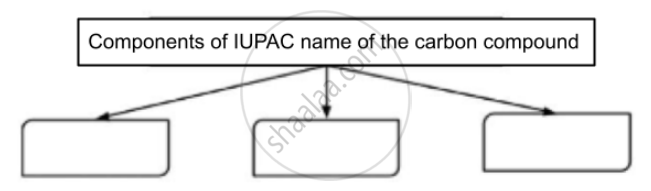Answer the Following | Q 8

Complete the following activity.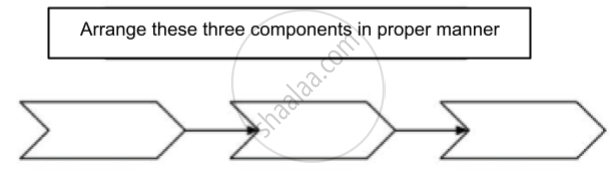Answer the Following | Q 9

Observe the structural formula and answer the following questions.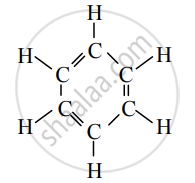1. Write the name of the given hydrocarbon.
2. The given hydrocarbon is included in which type of hydrocarbon?
3. What is the kind of compounds with the above characteristic structure called?
Answer the Following | Q 10

Complete the following chart by using examples given in brackets.

(isobutylene, cyclohexane, propane, cyclohexene, cyclopentane, benzene, propyne, isobutane, propene)

 Straight chain hydrocarbons Branched chain hydrocarbons Cyclic hydrocarbons
Answer the Following | Q 11.1

Complete the following activity.

Write the names of the hydrocarbons for the following structural formula.

(isobutylene, cyclohexane, propene, cyclohexene, cyclopentane, benzene, propyne, isobutane, propane)

 $\begin{array}{cc}\phantom{......}\ce{H}\phantom{...}\ce{H}\phantom{...}\ce{H}\phantom{..}\\\phantom{.....}|\phantom{....}|\phantom{....}|\\\ce{H - C - C = C}\\\phantom{.....}|\phantom{.........}|\\\phantom{.....}\ce{H}\phantom{........}\ce{H}\end{array}$
Answer the Following | Q 11.2

Complete the following activity.

Write the names of the hydrocarbons for the following structural formula.

(isobutylene, cyclohexane, propene, cyclohexene, cyclopentane, benzene, propyne, isobutane, propane)

 $\begin{array}{cc}\ce{H}\phantom{...}\ce{H}\phantom{...}\ce{H}\\|\phantom{....}|\phantom{....}|\\\ce{H - C - C - C - H}\\|\phantom{....}|\phantom{....}|\\\ce{H}\phantom{...}\ce{H}\phantom{...}\ce{H}\end{array}$
Answer the Following | Q 11.3

Complete the following activity.

Write the names of the hydrocarbons for the following structural formula.

(isobutylene, cyclohexane, propene, cyclohexene, cyclopentane, benzene, propyne, isobutane, propane)

 $\begin{array}{cc}\phantom{.........}\ce{H}\\\phantom{.........}|\\\ce{H - C ≡ C - C - H}\\\phantom{.........}|\\\phantom{.........}\ce{H}\end{array}$
Answer the Following | Q 11.4

Complete the following activity.

Write the names of the hydrocarbons for the following structural formula.

(isobutylene, cyclohexane, propene, cyclohexene, cyclopentane, benzene, propyne, isobutane, propane)

 $\begin{array}{cc}\phantom{..}\ce{H}\phantom{...}\ce{H}\phantom{...}\ce{H}\\\phantom{..}|\phantom{....}|\phantom{....}|\\\ce{H - C - C- C- H}\\\phantom{.}|\phantom{....}|\phantom{....}|\\\ce{H - C - H}\\|\\\ce{H}\end{array}$
Answer the Following | Q 11.5

Complete the following activity.

Write the names of the hydrocarbons for the following structural formula.

(isobutylene, cyclohexane, propene, cyclohexene, cyclopentane, benzene, propyne, isobutane, propane)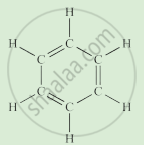Answer the Following | Q 11.6

Complete the following activity.

Write the names of the hydrocarbons for the following structural formula.

(isobutylene, cyclohexane, propene, cyclohexene, cyclopentane, benzene, propyne, isobutane, propane)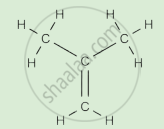Answer the Following | Q 11.7

Complete the following activity.

Write the names of the hydrocarbons for the following structural formula.

(isobutylene, cyclohexane, propene, cyclohexene, cyclopentane, benzene, propyne, isobutane, propane)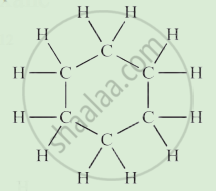Answer the Following | Q 11.8

Complete the following activity.

Write the names of the hydrocarbons for the following structural formula.

(isobutylene, cyclohexane, propene, cyclohexene, cyclopentane, benzene, propyne, isobutane, propane)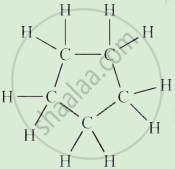Answer the Following | Q 11.9

Complete the following activity.

Write the names of the hydrocarbons for the following structural formula.

(isobutylene, cyclohexane, propene, cyclohexene, cyclopentane, benzene, propyne, isobutane, propane)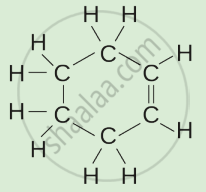### SCERT Maharashtra Question Bank solutions for 10th Standard SSC Science and Technology 1 Maharashtra State Board 2021 Chapter 9 Carbon compounds Answer the following questions

Answer the following questions | Q 1

Observe the figure and write the answers to the following questions.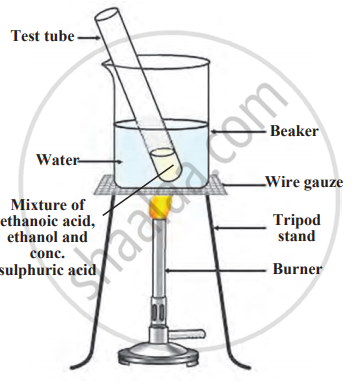1. Write the name of the reaction shown in the following figure.
2. Write the above chemical reaction in the form of a balanced equation.
3. Write the name of the product produced in the above reaction, write a use.
4. Write the name of the catalyst used in the above reaction.
Answer the following questions | Q 2

Write the answers to the questions by observing the following figure.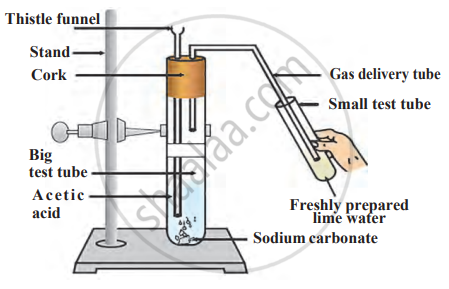1. Write the chemical reaction shown in the figure above in the form of a balanced equation.
2. Write the name of the gas coming out of the large test tube in the above chemical reaction.
3. Why do small bubbles appear in the small test tube?
4. What is the change in colour of lime water?

## Chapter 9: Carbon compounds

Choose the correct option.Find the correlation.Find odd one out.Answer in one sentence.Match the columnsRight or Wrong sentenceWrite an ExplanationWrite scientific reasonsSolve the following QuestionsDistinguish betweenWrite short notesAnswer the FollowingAnswer the following questions## SCERT Maharashtra Question Bank solutions for 10th Standard SSC Science and Technology 1 Maharashtra State Board 2021 chapter 9 - Carbon compounds

SCERT Maharashtra Question Bank solutions for 10th Standard SSC Science and Technology 1 Maharashtra State Board 2021 chapter 9 (Carbon compounds) include all questions with solution and detail explanation. This will clear students doubts about any question and improve application skills while preparing for board exams. The detailed, step-by-step solutions will help you understand the concepts better and clear your confusions, if any. Shaalaa.com has the Maharashtra State Board 10th Standard SSC Science and Technology 1 Maharashtra State Board 2021 solutions in a manner that help students grasp basic concepts better and faster.

Further, we at Shaalaa.com provide such solutions so that students can prepare for written exams. SCERT Maharashtra Question Bank textbook solutions can be a core help for self-study and acts as a perfect self-help guidance for students.

Concepts covered in 10th Standard SSC Science and Technology 1 Maharashtra State Board 2021 chapter 9 Carbon compounds are The Covalent Bond, Functional Groups in Carbon Compounds, Homologous Series of Carbon Compound, Nomenclature of Organic Compounds (IUPAC), IUPAC Nomenclature of Hydrocarbons, Ethanol, Ethanoic Acid, Carbon: a Versatile Element, Classification of Compounds of Carbon, Types of Covalent Bond, Formation of Covalent Bond, Hydrocarbons, Chemical Properties of Carbon Compound, Macro Molecules and Polymers, Special Features of Carbon, Chains, Branches and Rings of Carbon Compound, IUPAC Nomenclature of other classes.

Using SCERT Maharashtra Question Bank 10th Standard [इयत्ता १० वी] solutions Carbon compounds exercise by students are an easy way to prepare for the exams, as they involve solutions arranged chapter-wise also page wise. The questions involved in SCERT Maharashtra Question Bank Solutions are important questions that can be asked in the final exam. Maximum students of Maharashtra State Board 10th Standard [इयत्ता १० वी] prefer SCERT Maharashtra Question Bank Textbook Solutions to score more in exam.

Get the free view of chapter 9 Carbon compounds 10th Standard [इयत्ता १० वी] extra questions for 10th Standard SSC Science and Technology 1 Maharashtra State Board 2021 and can use Shaalaa.com to keep it handy for your exam preparation